# [原创图文] IEEE754标准 小数点后进制转换

8主题 0精华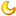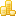学币
90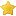荣耀
0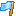rank
0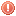违规
0发表于 2020-11-9 20:27:24 | 显示全部楼层 |阅读模式
 本帖最后由 鸦领主 于 2020-11-12 21:53 编辑 1.IEEE754（就是把一个小数用十六进制表示出来） 一个浮点数的组成分为三个部分 第1位是数符s s=1表示负数 s=0表示正数 第2-9位为移码E （双精度为2-12位） 第10-32位为尾数M （双精度为13-64位） 单精度浮点数偏移量127---1111 111 ^表示（“xx的xx次方”   比如2^3  2的3次方） 列：将3F 58 00 00转换成小数 →0011 1111 0101 1000 0000 0000 0000 0000 数符(0是正数，1是负数)：0正 阶码（移码-偏移量）：01111110-01111111=125-127=-1(十进制） 尾数（1.M）：.1011（隐含最高位1）→1.1011(二进制)→1.6875（十进制） 真值（尾数x2^-4）:1.6875x2^-1 =1.6875x0.5 =0.84375 IEEE754标准 小数点后进制转换列：将60.1转换成IEEE754 60.1(十进制）→11100.000110011001100110011001100(二进制) 数符（负数是1，正数是0）=0 尾数（1.M）：1.1100000110011001100110011001100（把1隐藏）=.1100000110011001100110011001100 阶码（小数点左移动一位代表阶码是1，右边移动一位代表阶码是-1）=5 移码（阶码+偏移量）=5+127=132(十进制）=10000100(二进制） →0100 0010 0110 0000 1100 1100 1100 1100（压缩到32位） →42 70 66 66H(H代表16进制) IEEE754标准 小数点后进制转换2.十进制小数转换二进制 如：0.625=（0.101） 0.625*2=1.25======取出整数部分1 0.25*2=0.5========取出整数部分0 0.5*2=1==========取出整数部分1 再如：0.7=（0.1 0110 0110...） 0.7*2=1.4========取出整数部分1 0.4*2=0.8========取出整数部分0 0.8*2=1.6========取出整数部分1 0.6*2=1.2========取出整数部分1 0.2*2=0.4========取出整数部分0 0.4*2=0.8========取出整数部分0 0.8*2=1.6========取出整数部分1 0.6*2=1.2========取出整数部分1 0.2*2=0.4========取出整数部分0 3.二进制小数转换十进制 如：0.110（二进制） 1/2=0.5 1/4=0.125 0/8=0 0.5+0.125=0.75 再如：0.101100110 1/2=0.5 0/4=0 1/8=0.125 1/16=0.0625 0/32=0 0/64=0 1/128=0.0078125 1/256=0.00390625 0/512=0 ...... 0.5+0.125+0.0625+0.0078125+0.00390625+......=0.7

1.如果您喜欢这篇帖子，请给作者点赞评分，点赞会增加帖子的热度，评分会给作者加学币。(评分不会扣掉您的积分，系统每天都会重置您的评分额度)。
2.回复帖子不仅是对作者的最好奖励，还可以获得学币奖励，请尊重作者的劳动成果，拒绝做伸手党！
3.发广告、灌水回复等违规行为一经发现直接禁言，如果本帖内容涉嫌违规，请点击论坛底部的举报反馈按钮，也可以在【投诉建议】板块发帖举报。